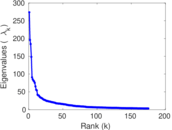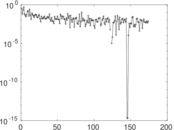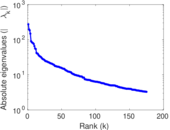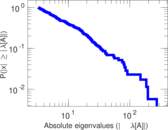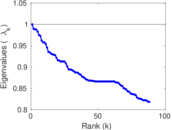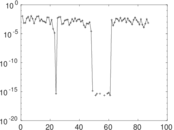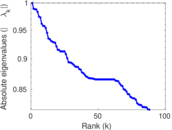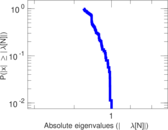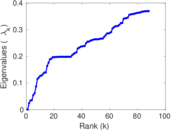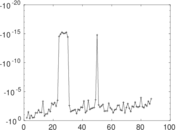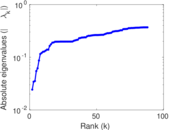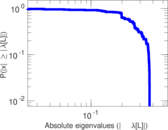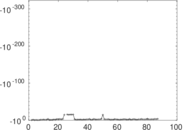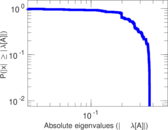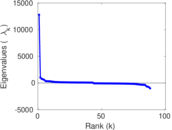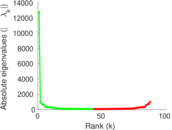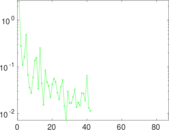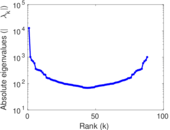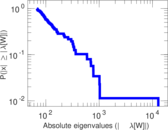# Wikipedia edits (bjn)

This is the bipartite edit network of the Banjar Wikipedia. It contains users and pages from the Banjar Wikipedia, connected by edit events. Each edge represents an edit. The dataset includes the timestamp of each edit.

 Code `bjn` Internal name `edit-bjnwiki` Name Wikipedia edits (bjn) Data source http://dumps.wikimedia.org/ AvailabilityDataset is available for download Consistency checkDataset passed all tests Category Authorship network Dataset timestamp 2017-10-20 Node meaning User, article Edge meaning Edit Network formatBipartite, undirected Edge typeUnweighted, multiple edges Temporal dataEdges are annotated with timestamps

## Statistics

 Size n = 12,801 Left size n1 = 822 Right size n2 = 11,979 Volume m = 53,330 Unique edge count m̿ = 31,918 Wedge count s = 42,525,885 Claw count z = 96,393,375,906 Cross count x = 191,906,509,589,813 Square count q = 17,197,376 4-Tour count T4 = 307,776,192 Maximum degree dmax = 10,747 Maximum left degree d1max = 10,747 Maximum right degree d2max = 210 Average degree d = 8.332 16 Average left degree d1 = 64.878 3 Average right degree d2 = 4.451 96 Fill p = 0.003 241 48 Average edge multiplicity m̃ = 1.670 84 Size of LCC N = 12,367 Diameter δ = 12 50-Percentile effective diameter δ0.5 = 1.975 61 90-Percentile effective diameter δ0.9 = 3.842 94 Median distance δM = 2 Mean distance δm = 2.968 40 Gini coefficient G = 0.835 574 Balanced inequality ratio P = 0.137 905 Left balanced inequality ratio P1 = 0.070 673 2 Right balanced inequality ratio P2 = 0.203 694 Relative edge distribution entropy Her = 0.730 768 Power law exponent γ = 3.080 92 Tail power law exponent γt = 2.111 00 Tail power law exponent with p γ3 = 2.111 00 p-value p = 0.000 00 Left tail power law exponent with p γ3,1 = 1.681 00 Left p-value p1 = 0.000 00 Right tail power law exponent with p γ3,2 = 5.151 00 Right p-value p2 = 0.000 00 Degree assortativity ρ = −0.497 948 Degree assortativity p-value pρ = 0.000 00 Spectral norm α = 273.831 Algebraic connectivity a = 0.024 248 7 Spectral separation |λ1[A] / λ2[A]| = 1.396 74 Controllability C = 11,260 Relative controllability Cr = 0.882 722

## Plots

### Fruchterman–Reingold graph drawing### Degree distribution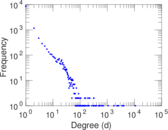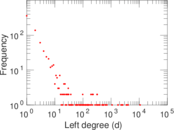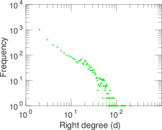### Cumulative degree distribution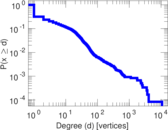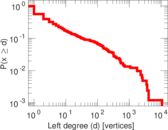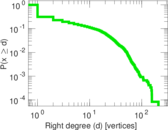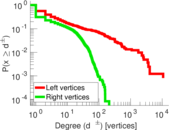### Lorenz curve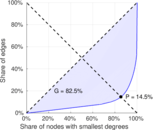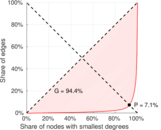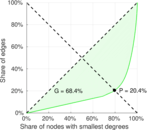### Spectral distribution of the adjacency matrix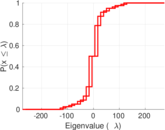### Spectral distribution of the normalized adjacency matrix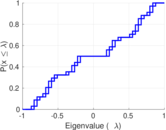### Spectral distribution of the Laplacian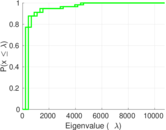### Spectral graph drawing based on the adjacency matrix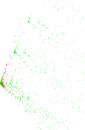### Spectral graph drawing based on the Laplacian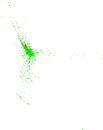### Spectral graph drawing based on the normalized adjacency matrix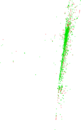### Degree assortativity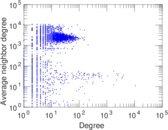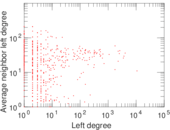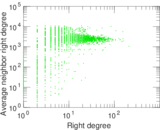### Zipf plot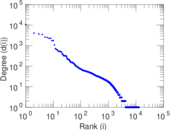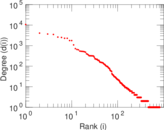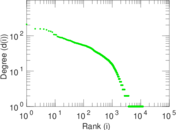### Hop distribution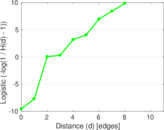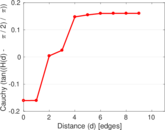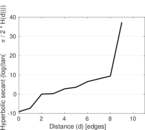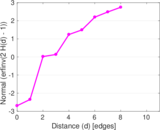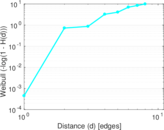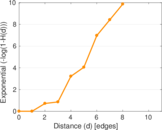### Double Laplacian graph drawing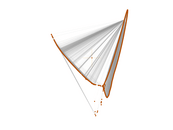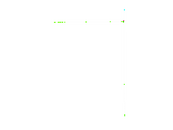### Delaunay graph drawing### Edge weight/multiplicity distribution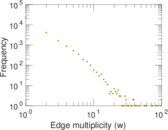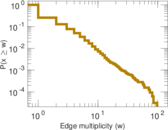### Temporal distribution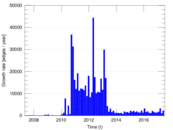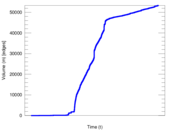### Temporal hop distribution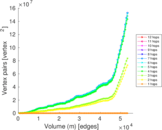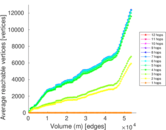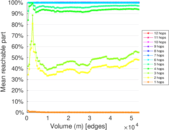### Diameter/density evolution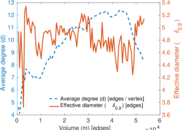### Matrix decompositions plots